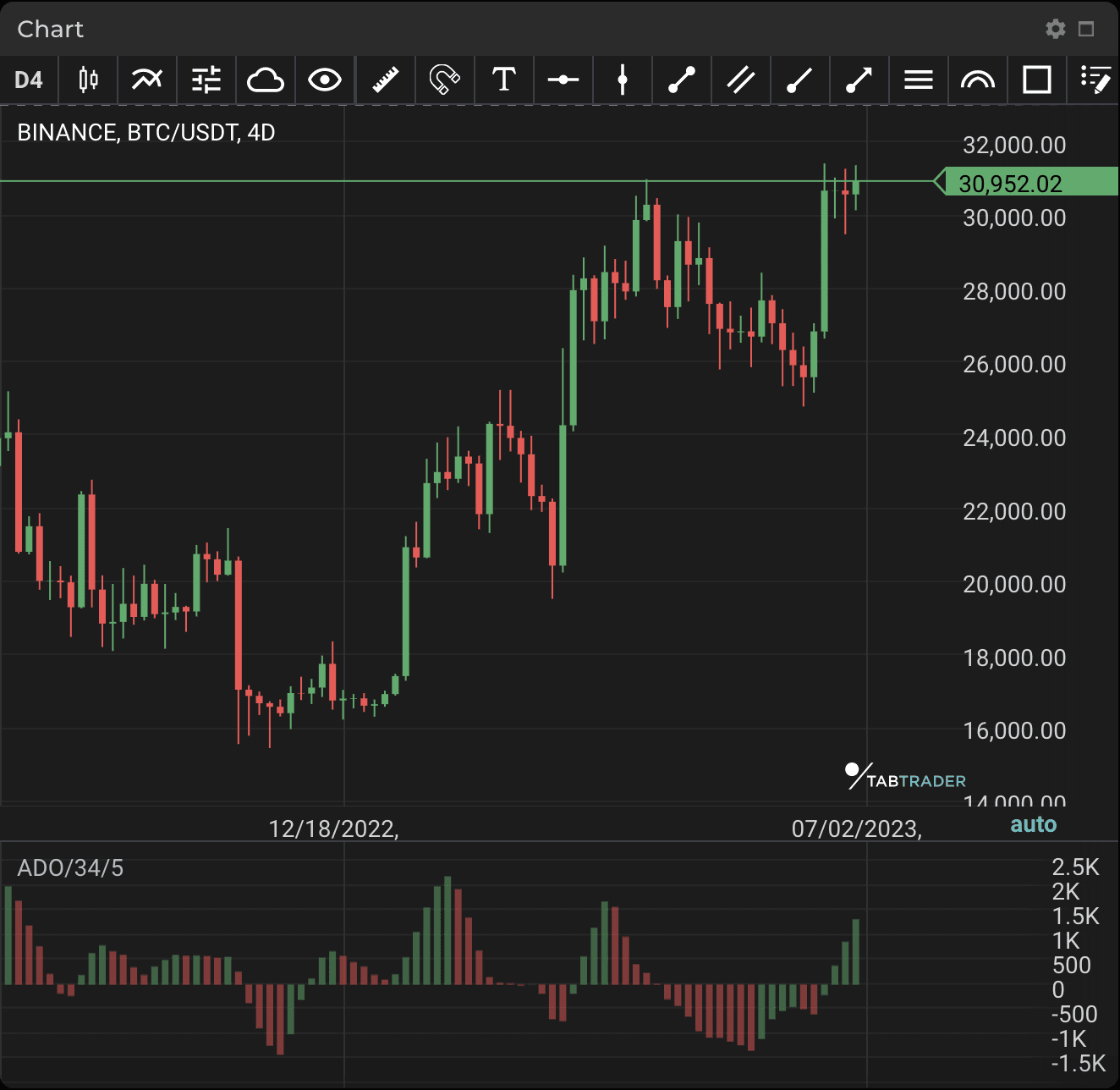# Accelerator Decelerator Oscillator

Publication date is

The Accelerator Decelerator Oscillator (ADO) measures acceleration and deceleration of current momentum. This indicator tends to change direction before any changes in momentum, which in turn will change direction before price. If you find an early warning in momentum acceleration or deceleration, it may give you a certain trading advantage.### Calculation

ADO (referred to in the calculations below as the Accelerator Oscillator (AC)), is the difference between the Awesome Oscillator (AO) and the AO 5-period moving average.

MEDIAN PRICE = (HIGH + LOW) / 2

AO = SMA (MEDIAN PRICE, 5) - SMA (MEDIAN PRICE, 34)

AC = AO - SMA (AO, 5)

Where: MEDIAN PRICE — median price;

HIGH — the highest price of the bar;

LOW — the lowest price of the bar;

SMA — Simple Moving Average;

AC — Accelerator Oscillator (Accelerator Decelerator Oscillator);

AO — Awesome Oscillator.

Looking to get started in crypto trading?

Try TabTrader on mobile or web!